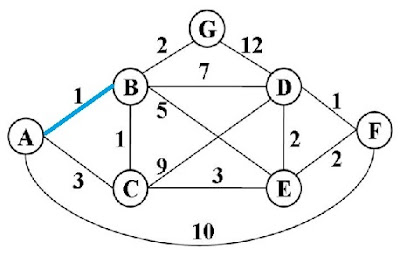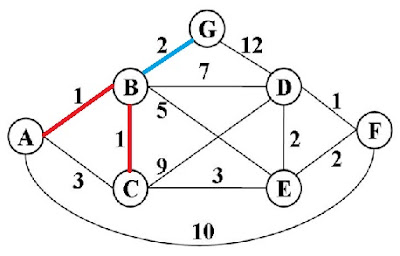Please visit, subscribe and share 10 Minutes Lectures in Computer Science
Showing posts with label Data Structures. Show all posts
Showing posts with label Data Structures. Show all posts

# Data structures and algorithms multiple choice questions with answers, important interview questions in data structures, data structures questions for entrance exams, frequently asked questions in data structures and algorithms, GATE questions in data structures with explained answers

## SET 11

1. Consider a situation where the data to be sorted is too big to fit in memory, so most of it is on disk. Which of the following is the best algorithm to sort such data?

a) Insertion sort

b) Heap sort

c) Merge sort

d) All of the above

2. Consider a large data set, where all the data has only one of about 10 values for sorting purposes (e.g., the data is records of employees of an organization where the legal age range is 25 to 60 and the sort is by age in years). Which of the following is the best algorithm to sort such data?

a) Insertion sort

b) Bucket sort

c) Merge sort

d) Heap sort

3. Given a dataset, we would like to sort the first k smallest (k is much smaller than the size of the dataset) in ascending order. Which of the following is the best algorithm to sort such data?

a) Insertion sort

b) Selection sort

c) Merge sort

d) Bucket sort

4. If h is any hashing function chosen from universal collection of hash functions and is used to hash n keys in to a table of size m, where n<=m, the expected number of collisions involving a particular key x is

a) <m

b) <n

c) < half of n

d) <1

5. Which among the following is a technique of direct search?

a) Binary search

b) Linear search

c) Hash search

d) All of the above

*************************

# Dijkstra's algorithm in data structures, single source shortest path algorithm solved exercise

Question: Consider the given undirected weighted graph. Use Dijkstra’s algorithm to calculate the single-source shortest paths from A to every other vertex.

Solution:

 Vertex Known Cost from source A Minimum cost to reach next vertex Next vertex chosen Visited vertices A (source) Y 0 - A From A, we can go to either B, or C, or F B Y 1 Choose next vertex as follows; minimum(AB, AC, AF) = min(1, 3, 10) = 1 Choose B (cost = 1) as the next vertex. B AB C Y 3 F Y 10 Vertices visitedFrom B, we can go to C, D, E, or G and from A we can reach C directly C Y AB+BC = 1+1 = 2 Min(ABC, ABD, ABE, ABG, AC) = min(2, 8, 6, 3, 3) = 2 Choose C (cost = 2) as the next vertex. C ABC D Y AB+BD = 1+7 = 8 E Y AB+BE = 1+5 = 6 G Y AB + BG = 1+2 = 3 C Y AC = 3 Vertices visitedFrom C, we can reach D or E and from B we can reach G D Y ABC+CD = 2+9 = 11 Min(ABCD, ABCE, ABG) = min(11, 5, 3) = 3 Choose G (cost = 3) as the next vertex. G ABCG E Y ABC+CE = 2+3 = 5 G Y AB+BG = 1+2 = 3 Vertices visitedFrom G, we can go to D and from C we can go to D or E D Y ABG+GD = 3+12 = 15 Min(ABGD, ABCE, ABCD) = min(15, 5, 11) = 5 Choose E (cost = 5) as the next vertex. E ABCEG E Y ABC+CE = 2+3 = 5 D Y ABC+CD = 2+9 = 11 Vertices visitedNext we can reach D or F D Y ABG+GD = 3+12 = 15 Min(ABGD, ABD, ABCED, ABCD, ABCEF, AF) = min(15, 8, 7, 11, 7, 10) = 7   Choose D or F (cost = 7) as the next vertex.   After D, we can choose F (cost = 7) as the next vertex. D F ABCDEFG D Y AB+BD = 1+7 = 8 D Y ABCE+ED = 5+2 = 7 D Y ABC+CD = 2+9 = 11 F Y ABCE+EF = 5+2 = 7 F Y AF = 10

The shortest path will be;

From this graph, we can get the shortest path from single source (A in this case) to any other vertices. For instance, the shortest path from A to D is ABCED with the cost 7 (AB+BC+CE+ED). The shortest path from A to G is ABG with the cost 3 (AB+BG). The shortest path from A to C is ABC with the cost 2 (AB+BC) etc.

****************

## Featured Content

### Multiple choice questions in Natural Language Processing Home

MCQ in Natural Language Processing, Quiz questions with answers in NLP, Top interview questions in NLP with answers Multiple Choice Que...

data recovery